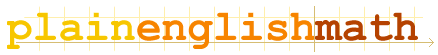You are here: MathematicsMatrices → Matrices

Matrices

Introduction
What is a matrix?

Matrix algebra

Matrix operations
How to add, subtract and multiply matrices